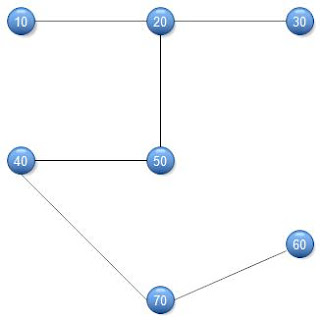Don't miss
Showing posts with label Graph. Show all posts
Showing posts with label Graph. Show all posts

Prim's Algorithm

Prim's Algorithm are those algorithm which is also used to find shortest minimum spamming tree(weight of edges are minimal) like Kruskal's Algorithm.But its way of creation is different.This Algorithm is used in c,c++,java,c# etc.

Example:-

Step 1:-Arrange the edges in increasing order of weight.

Step 2:-Choose the nearest neighbour and add.

Step 3:-Repeat step 2 until it has n-1 vertex and non cyclic.

Step 4:-Exit code.

Solution:-Prims Algorithm(in graph)

Kruskal's Algorithm

Kruskal's Algorithm are those algorithm which is used to find shortest minimum spamming tree(weight of edges are minimal).This Algorithm is used in c,c++,java,c# etc.

Example:-

Step 1:-Arrange the vertex in increasing order of weight.

Step 2:-Add the edges until it becomes cyclic (n-1 edges).

Step 3:-Exit code.

Solution:-Kruskal Algorithm(in graph)

Breath First Search

Breath First Search:-Breath First Search is a traversal technique comes in a Traverse Scheme of vertex's.This technique used in c,c++,java,C# etc.

BFS Algorithm:-

Now I discuss this algorithm in step wise. Step 1:-The Starting Vertex is A,initialise all vertex to ready state i.e. status=1.

Step 2:-Put A in Queue and change the status to waiting state i.e. status=2.

Step 3:-Repeat Step 4 & 5(until queue=empty).

Step 4:-Remove the top vertex and change the state to process state i.e. status=3.

Step 5:-(a)If status=1 then add vertex to rear and change the state into waiting  state.

(b)If status=2 then ignored.

(c)If status=3 then ignored.

Step 6:-Exit.

Example:-

NULL    A
A    B,C,D
B    C,D,E,F
C    D,E,F
D    E,F
E    F,G
F    G
G    H
H    NULL

Depth First Search

Depth First Search:-Depth First Search is a major technique which comes in a Traverse scheme of vertex's.This technique is used in c,c++,java,C# etc.

DFS Algorithm:-

Now I discuss this algorithm in step wise. Step 1:-The Starting Vertex is A,initialise all vertex to ready state i.e. status=1.

Step 2:-Push A to stack and change the status to waiting state i.e. status=2.

Step 3:-Repeat Step 4 & 5(until stack=empty).

Step 4:-Pop the top vertex and change the state to process state i.e. status=3.

Step 5:-(a)If status=1 then Push vertex to stack and change the state into waiting  state.

(b)If status=2 then Delete vertex from stack and change the state into Process state.

(c)If status=3 then ignored.

Step 6:-Exit.

Example:-DFS Algorithm(Tree)

NULL     A
A     B,C,D
B     E,F,C,D
E     F,G,C,D
F     C,G,D
C     G,D
G     H,D
H     D
D     NULL

ABEFCFEGHEBAA

Graph(Data Structure)

Graph is a collection of ordered pair of sets including vertex and edges(V,E).Graph is a non-linear data structure and provide many to many(M:M) relationship.We used different-2 operation to finding the path of nodes and shortest path of nodes like Depth First Search(DFS),Breath First Search(BST) and Dijkstra's Algorithm,Kruskal's Algorithm respectively.Graph used in c,c++,java,c# etc.

Example of Graph:-

Type of Graph or Application or Theory of Graph:-

1)Undirected Graph:-Undirected Graph are those graph whose edges are undirected to each other(no direction).

2)Directed Graph:-Directed Graph are those graph whose edges are directed(has a direction).

3)Mixed Graph:-Mixed Graph are those Graph in which some edges are directed and some are not.

4)Simple Graph:-Simple Graph are those graph which has no parallel(//) edges.

5)Multi-Graph:-Multi-Graph are those graph which either // edges or self edges.

6)Isolated Graph:-Isolated Graph are those graph whose degree of vertex is zero or 0.

7)Connected Graph:-Connected Graph are those graph whose all vertex are connected to each other.

8)Disconnected Graph:-Disconnected Graph are those graph whose all vertex are not connects each other nodes.

9)Weakly Connected Graph(Di Graph):-Di Graph are those graph whose nodes are traverse to reach any node.

10)Strongly Connected Graph:-Strongly Connected Graph are those graph whose nodes are not traverse to reach any node.

11)Null Graph:-Null Graph are those graph which contains only isolated vertex.

12)Planner Graph:-Planner Graph are those graph which has no cross edge.

13)Tree(Graph):-Tree is also called as graph which has n-1 edges.

14)Isomorphic Graph:-Isomorphic Graph are those graph which has same number of vertex,edges and degree but its structure are different.